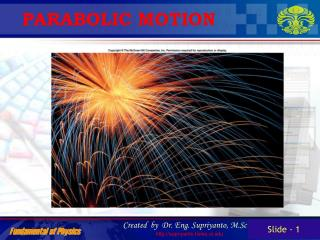DownloadDownload PresentationPARABOLIC MOTION

# PARABOLIC MOTION

Download Presentation## PARABOLIC MOTION

- - - - - - - - - - - - - - - - - - - - - - - - - - - E N D - - - - - - - - - - - - - - - - - - - - - - - - - - -
##### Presentation Transcript

1. PARABOLIC MOTION

2. Describing parabolic motion Parabolic motion refers to the motion of an object that is thrown, or projected, into the air at an angle. Parabolic motion is a combination of horizontal motion with constant horizontal velocity and vertical motion with a constant downward acceleration due to gravity. The vertical motion of a projected object is independent of its horizontal motion. The one common variable between the horizontal and vertical motions is time.

3. Parabolic motion Velocities vector of horizontal and vertical motion

4. Parabolic motion • Horizontal • Motion of a ball rolling freely along a level surface • Horizontal velocity is ALWAYS constant • Vertical • Motion of a freely falling object • Force due to gravity • Vertical component of velocity changes with time • Parabolic • Path traced by an object accelerating only in the vertical direction while moving at constant horizontal velocity

5. Parabolic motion Horizontal and vertical motion

6. Parabolic motion Time of flight is determined by vertical motion

7. The bullet motion

8. Angle for maximum distance

9. Angle for maximum distance

10. Projectile motion Horizontal component of velocity is constant over entire path! vx = v0x= v0cosa No acceleration in horizontal direction

11. Projectile motion Vertical component of velocity constantly changing due to gravitational acceleration in -y direction v0y --> 0 -> -v0y v0y = v0sina

12. Projectile motion At the top of the trajectory: t = 1/2 of total time x = 1/2 of total horizontal range

13. Projectile motion Horizontal motion of projectile: vx = v0cos a= constant Dx = v0xt = (v0cos a)t

14. Projectile motion Vertical motion of projectile: vy = v0sin a - gt Dy = (v0sin a)t - 1/2gt2 vy2 = (v0sin a)2 - 2gDy

15. Projectile motion Combined 2D motion: v = (vx2 + vy2)1/2 tanq = vy/vx q = tan-1(vy/vx) -90 < q < 90

16. Simulation#1

17. Simulation#2

18. Simulation#3

19. Exercise#1

20. Summary • A projectile is a body in free fall that is affect only by • gravity and air resistance. • Projectile motion is analyzed in terms of its horizontal and • vertical components. • Vertical is affect by gravity • Factors that determine the height & distance of a projectile • are; projection angle, projection speed, and relative • projection height • The equation constant acceleration can be used to • quantitatively analyze projectile motion.

21. Exercise #2: • A batter hits a ball at 35 with a velocity of 32 m/s. • How high did the ball go? • H = 17 m • How long was the ball in the air? • t = 3.8 s • How far did the ball go? • x = 98 m

22. Exercise #2: • 2. While driving down a road a bad guy shoots a bullet • straight up into the air. If there was no air resistance • where would the bullet land – in front, behind, or on him? • If air resistance present, bullet slows and lands behind. • No air resistance, the Vx doesn’t change and bullet lands on him.

23. Exercise #2: 3. A truck (v = 11.2 m/s) turned a corner too sharp and lost part of the load. A falling box will break if it hits the ground with a velocity greater than 15 m/s. The height of the truck bed is 1.5 m. Will the box break? v = 12 m/s, No it doesn’t break

24. Exercise #2: 4. A meatball with v = 5.0 m/s rolls off a 1.0 m high table. How long does it take to hit the floor? t = 0.45 s What was the velocity when it hit? v = 6.7 m/s @ 42°